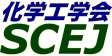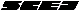English page## \$B9V1i?=9~\$N8!:w(B

\$B!&=jB0N,>N!W!"!V%-!<%o!<%I!W\$K\$D\$\$\$F8!:w\$G\$-\$^\$9!#(B

### \$B8!:w>r7o(B

\$B\$K(B \$B\$H\$\$\$&(B \$B\$r4^\$`(B
\$B\$?\$@\$7!"(B \$B7o\$^\$G\$7\$+I=<(\$7\$J\$\$(B

\$B%"%k%U%!%Y%C%H\$NBgJ8;z\$H>.J8;z\$O6hJL\$5\$l\$^\$;\$s!#(B
Perl \$B\$N@55,I=8=\$rMQ\$\$\$?8!:w\$b2DG=\$G\$9!#(B Perl \$B\$N@55,I=8=\$G\$O!"(B . ^ \$ \ * + ? [ ] ( ) | \$B\$N3FJ8;z\$K\$O(B \$BFCl9g\$O(B \$B\$=\$NJ8;z\$NA0\$K(B \ \$B\$rIU\$1\$F\$/\$@\$5\$\$!#(B

\$B2=3X9)3X2q(B \$BBh(B44\$B2s=)5(Bg2q(B(C) 2012 \$B8x1W
Most recent update: 2012-06-26 18:15:49
For more information contact \$B2=3X9)3X2qElKL;YIt(B \$BBh(B44\$B2s=)5(Bg2q(B \$BLd\$\$9g\$;78(B
E-mail: inquiry-44fwww3.scej.org
This page was generated byeasp 2.28; mknote 2.28X (C)1999-2012 kawase